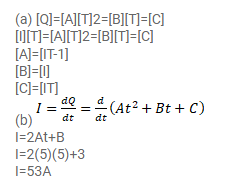# The amount of charge passed in time t throughQuestion:

The amount of charge passed in time $t$ through a cross-section of a wire is $Q(t)=A t^{2}+B t+C .$

(a) Write the dimensional formulae for A, B and C.

(b) If the numerical value of $A, B$ and $C$ are 5,3 and 1 respectively in SI units, find the value of current at $t=5 s$.

Solution: